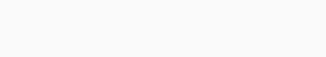# Calculate the Median Absolute Deviation in Python

This tutorial is intended to help you calculate the median absolute deviation in Python. Median absolute deviation commonly known as (MAD) is an indicator of variability, the median absolute deviation (MAD), measures the spread of a data set. The formula used for this method is given below:The calculation of the median absolute deviation can be determined with the help of several methods, some of which are covered in this tutorial.

## Method 1. Using SciPy to calculate median absolute deviation

Using the Python SciPy library, the median absolute deviation can be calculated based on an array of values. First, import the required libraries and make a list of numbers, then calculate the median using `median_abs_deviation()` of the SciPy library. For a better understanding, the code is given below.

```# import the required libraries
from scipy.stats import median_abs_deviation
#creating a list of numbers
numbers = [56, 70, 85, 69, 29, 32, 16, 32, 42, 24, 33, 28, 86, 64, 100, 19, 100, 58, 50, 61, 39, 78, 5, 23, 64]
median_absolute_deviation = median_abs_deviation(numbers)
#print the median absolute deviation
print(median_absolute_deviation)

```

Output:

`20.0`

## Method 2. Using Pandas

Additionally, we use the Pandas library in Python to calculate the median absolute deviation. It is useful to use the Pandas library to compute the median absolute deviation in a tabular datasheet for multiple columns. The apply method is a convenient way to calculate median absolute deviation in Pandas since the function does not exist. As soon as you import the Pandas and SciPy libraries, you create the data frame of the given list, and at last, you print it out by applying the apply method. For reference, the code of this method is given below.

```# import the needed libraries
import pandas as pd
from scipy.stats import median_abs_deviation
#create the list of numbers
numbers = [56, 70, 85, 69, 29, 32, 16, 32, 42, 24, 33, 28, 86, 64, 100, 19, 100, 58, 50, 61, 39, 78, 5, 23,64]
#convert the list into the dataframe
df = pd.DataFrame(numbers, columns=['Numbers'])
#print the mean absolute deviation
print(df[['Numbers']].apply(median_abs_deviation))```

Output:

```Numbers 20.0
dtype: float64
```